# 4.3 线性判别分析¶

• 采用高斯密度的线性和二次判别分析
• 更加灵活的混合的高斯密度，允许非线性判别边界（6.8 节
• 对每个类别密度进行一般的非参数密度估计，允许最大的灵活性（6.6.2 节
• 朴素贝叶斯(Naive Bayes) 模型是上个情形的变种，并且假设每个类密度是边缘密度的乘积，这也就是，假设输入在每一类中都是条件独立的（6.6.3节

weiya 注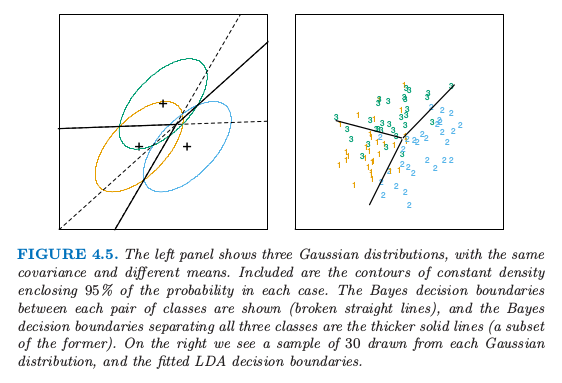weiya 注：重现图 4.5

• $\hat \pi_k=N_k/N$，其中 $N_k$ 是第 $k$ 类观测值的个数；
• $\hat\mu_k=\sum_{g_i=k}x_i/N_k$；
• $\hat{\boldsymbol\Sigma}=\sum_{k=1}^K\sum_{g_i=k}(x_i-\hat\mu_k)(x_i-\hat\mu_k)^T/(N-K)$．

weiya 注：Recall

weiya 注：Ex. 4.2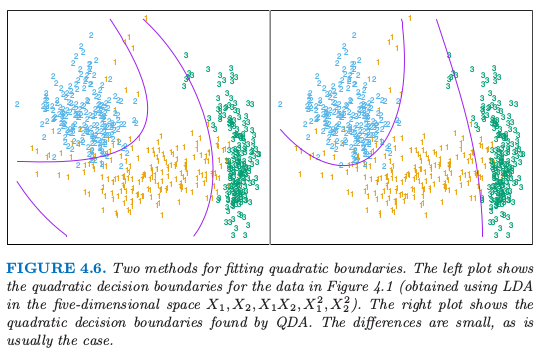QDA 的估计类似 LDA 的估计，除了协方差矩阵必须要按每一类来估计．当 $p$ 很大这意味着系数有显著性的增长．因为判别边界是系数密度的函数，参数的个数必须要考虑．对于 LDA，似乎有 $(K-1)\times(p+1)$ 个参数，因为我们仅仅需要判别函数之间的不同 $\delta_k(x)-\delta_K(x)$，其中 $K$ 是一些预先选好的类别（这里我们已经选了最后一类），每个差异需要 $p+1$ 个参数．对于 QDA 类似地，我们会有 $(K-1)\times \{p(p+3)/2+1\}$ 个参数．LDA 和 QDA 在非常大以及离散的数据集的分类上面表现得很好．举个例子，在 STATLOG 项目中 (Michie et al. 19943) LDA 在 7 个数据集（总共 22 个数据集）中的表现排前三名，QDA 在四个数据集中排前三名，对于 10 个数据集两种方法的其中一种排前三名．两种方法都被广泛运用，整本书集中讨论 LDA．在各种外来方法风靡一时的今天，我们总是会有两种简单的方法可以使用．为什么 LDA 和 QDA 有那么好的效果？原因不可能是数据近似服从高斯分布，对于 LDA 协方差矩阵也不可能近似相等．很可能的一个原因是数据仅仅可以支持简单的判别边界比如线性和二次，并且通过高斯模型给出的估计是稳定的，这是一个偏差与方差之间的权衡——我们可以忍受线性判别边界的偏差因为它可以通过用比其它方法更低的方差来弥补．这个论点对于 QDA 更是不可想象，因为它自身有许多的参数，尽管或许比非参估计的参数要少．

## 正则化判别分析¶

Friedman (1989)2 提出 LDA 和 QDA 之间的一个权衡，使得 QDA 的协方差阵向 LDA 中的共同协方差阵收缩．这些方法非常类似岭回归．正则化协方差矩阵有如下形式 其中，$\hat\Sigma$ 是和 LDA 一样用的联合协方差矩阵．这里 $\alpha\in[0,1]$ 允许在 LDA 和 QDA 之间连续变化的模型，而且需要指定．实际中，$\alpha$ 可以基于在验证数据的表现上进行选择，或者通过交叉验证．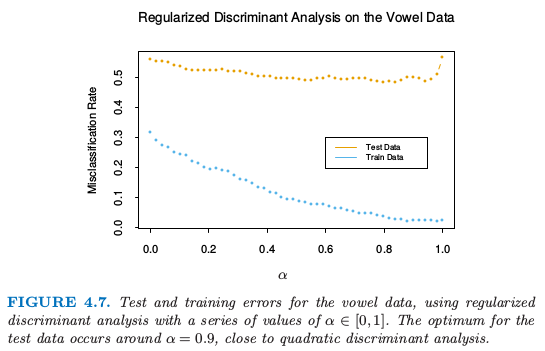## LDA 的计算¶

• $(x-\hat\mu_k)^T\hat\ssigma_k^{-1}(x-\hat\mu_k)=[U_k^T(x-\hat\mu_k)]^TD_k^{-1}[U_k^T(x-\hat\mu_k)]$
• $\log\vert \hat\ssigma_k\vert=\sum_{\ell}\log d_{k\ell}$

• 对数据关于协方差矩阵 $\hat{\boldsymbol\Sigma}$ 球面化：$X^*\leftarrow \mathbf D^{-\frac{1}{2}}\mathbf U^T\mathbf X$，其中 $\hat{\boldsymbol\Sigma}=\mathbf U\mathbf D\mathbf U^T$．$X^*$ 的共同协方差矩阵变为单位阵．
• 考虑类别先验概率 $\pi_k$ 的影响，在变换后的空间里面分到最近的类别形心．

## 降维线性判别分析¶

weiya 注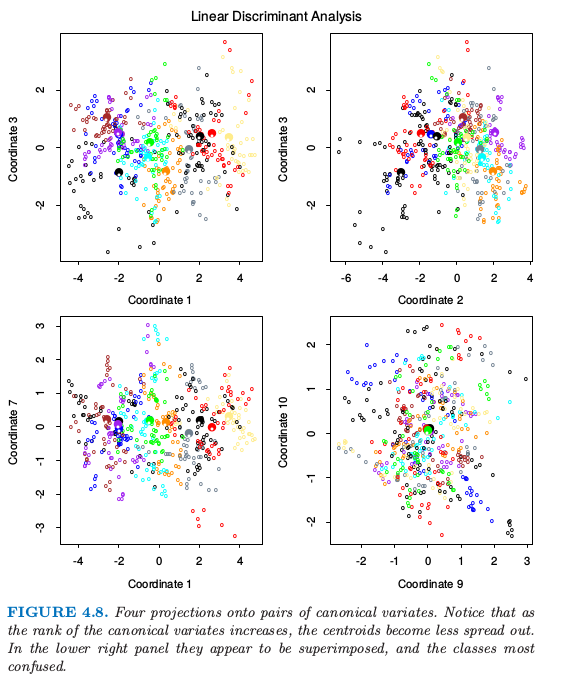• 计算 $K\times p$ 的类别形心矩阵 $\mathbf M$ 以及共同协方差矩阵 $\mathbf W$（组内 (within-class) 协方差）
• 使用 $\mathbf W$ 特征值分解计算 $\mathbf M^*=\mathbf M\mathbf W^{-\frac{1}{2}}$
• 计算 $\mathbf M^*$ 的协方差矩阵 $\mathbf B^*$，（$\mathbf B$ 是 组间 (between-class) 协方差），以及特征值分解 $\mathbf B^*=\mathbf V^*\mathbf D_B{\mathbf V^*}^T$．$\mathbf V^*$ 的列 $v_\ell^*$ 从第一个到最后一个依次定义了最优子空间的坐标．

weiya 注：

Fisher 通过不同的方式得到这个分解，完全没有引用高斯分布．他提出下面的问题：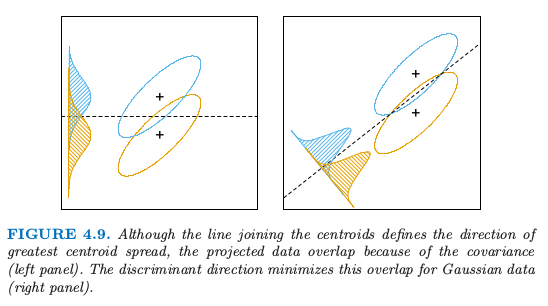$Z$ 的组间方差为 $a^T\mathbf Ba$，而组内方差为 $a^T\mathbf Wa$，$\mathbf W$ 是很早定义的，$\mathbf B$ 是类别形心矩阵 $\mathbf M$ 的协方差矩阵．注意到 $\mathbf {B+W=T}$，其中 $\mathbf T$ 是 $\mathbf X$ 的总协方差矩阵，忽略掉了类别信息．

weiya 注：

Fisher 问题因此等价于最大化 Rayleigh quotient, 或者等价地， 这是一个一般化的特征值问题，$a$ 是由 $\mathbf W^{-1}\mathbf B$ 的最大特征值给出．不难证明（练习 4.1）最优 $a_1$ 等于上面定义的 $v_1$．类似地，可以找到下一个方向 $a_2$，在 $W$ 中与 $a_1$ 正交，使得 $a_2^TBa_2/a_2^TWa_2$ 最大化；解为 $a_2=v_2$，其余类似．$a_\ell$ 被称作 判别坐标 (discriminant coordinates)，不会与判别函数相混淆．他们也被称作 典则变量 (canonical variables)，因为这些结果的一个变形是通过在预测变量矩阵 $X$ 上对指示响应矩阵 $Y$ 进行典则相关分析得到的．这一点将在 12.5 节继续讨论．

weiya 注：Ex. 4.1

• 有相同的协方差矩阵的高斯分类导出线性判别边界．分类可以通过对数据关于 $\mathbf W$ 球面化得到，并且划分到球空间的最近形心内（矫正因子 $\log\pi_k$）
• 因为只计算了到形心的相对距离，所以可以把数据局限于在球空间的形心张成的子空间．
• 子空间可以进一步分解为关于形心分离的最优子空间．这个分解与 Fisher 的分解相同．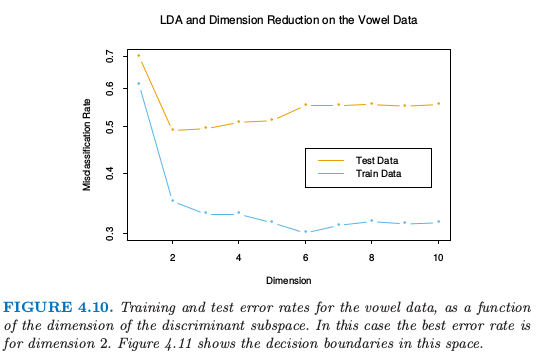Fisher 降秩判别分析 (RDA) 和指示响应矩阵回归之间存在着紧密的联系．事实表明 LDA 意味着 $\hat{\mathbf Y}^T\mathbf Y$ 的特征值分解后进行回归．在两个类的情形下，存在一个单判别变量，乘上一个标量后能与 $\hat{\mathbf Y}$ 的某一列相等．这些联系将在第 12 章中讨论．一个相关的事实是先将原始的预测变量 $\mathbf X$ 转换为 $\hat{\mathbf Y}$，然后用 $\hat{\mathbf Y}$ 做 LDA 与在原空间中做 LDA 是相同的（练习 4.3）．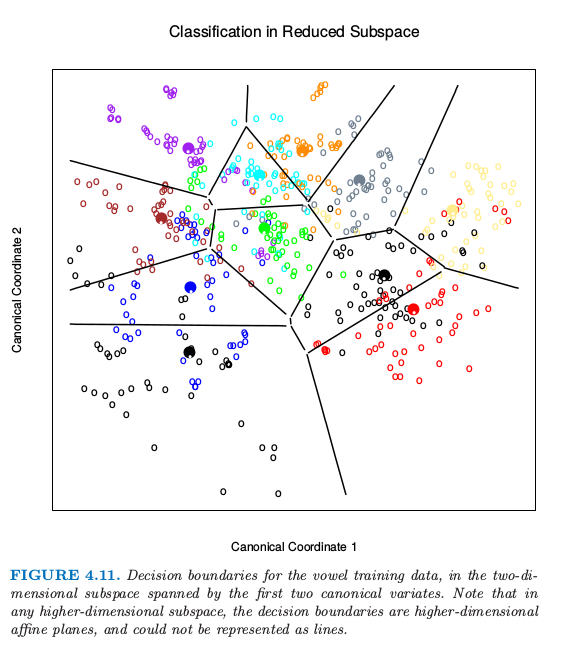1. Hastie, T., Tibshirani, R. and Buja, A. (1994). Flexible discriminant analysis by optimal scoring, Journal of the American Statistical Association 89: 1255–1270.

2. Friedman, J. (1989). Regularized discriminant analysis, Journal of the American Statistical Association 84: 165–175.

3. Michie, D., Spiegelhalter, D. and Taylor, C. (eds) (1994). Machine Learning, Neural and Statistical Classification, Ellis Horwood Series in Artificial Intelligence, Ellis Horwood.